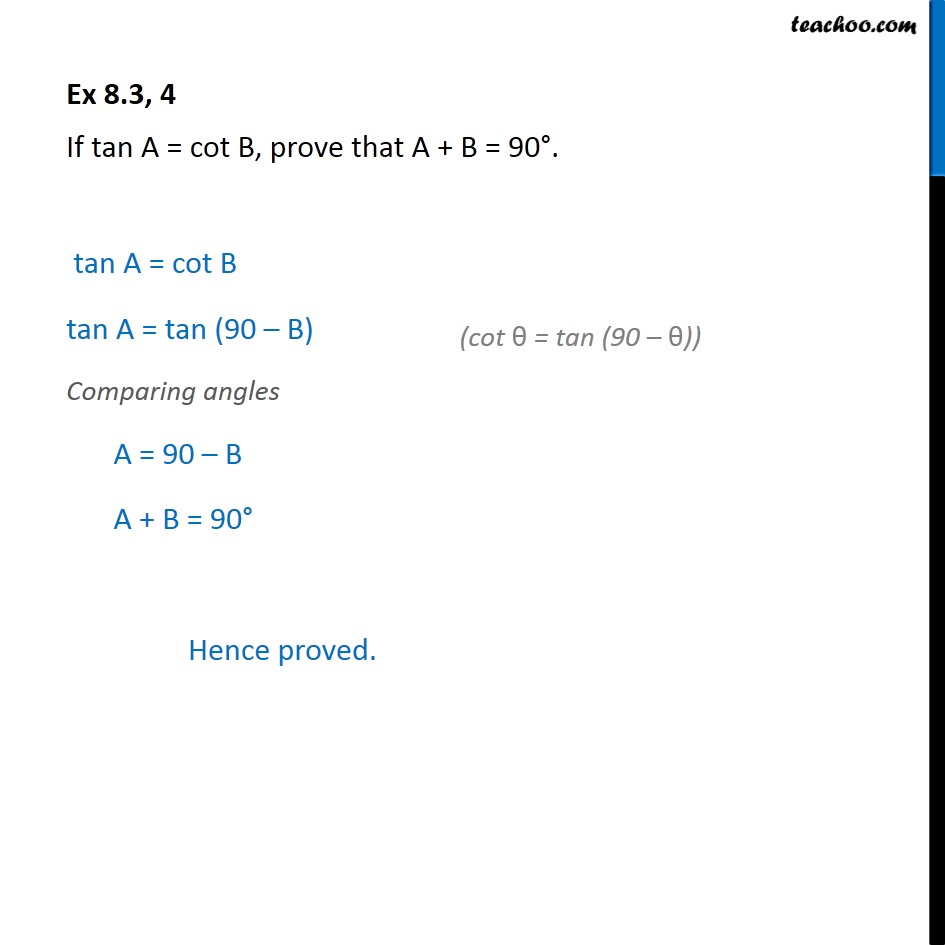1. Chapter 8 Class 10 Introduction to Trignometry (Term 1)
2. Concept wise
3. Trignometric ratios of complementry angles

Transcript

Ex 8.3, 4 If tan A = cot B, prove that A + B = 90 . Given tan A = cot B To solve this we should know relation between tan and cot Now, We know that cot θ = tan (90 - θ) Putting value of cot B tan A = tan (90 - B) Comparing angles A = 90 - B A + B = 90 Hence proved.

Trignometric ratios of complementry angles